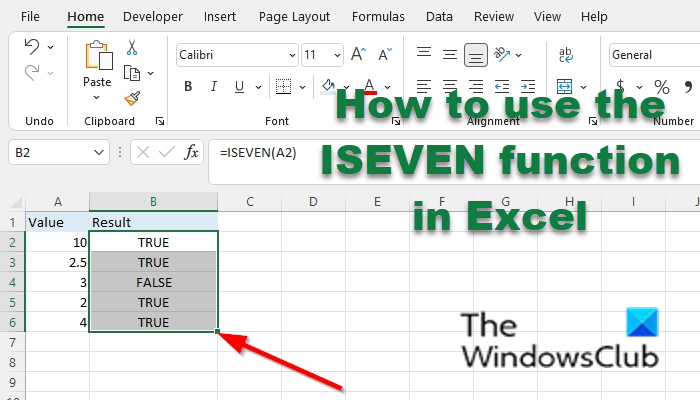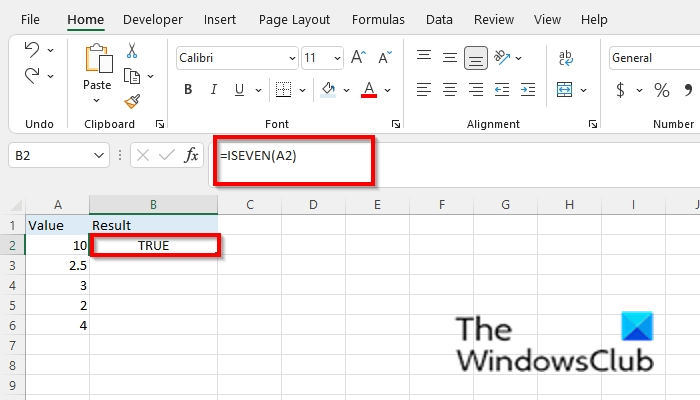# How to use the ISEVEN function in Excel

In Microsoft Excel, the ISEVEN function is an information function, and its purpose is to return True if the number is even and returns false if the number is odd. Information functions in Excel return information about the formatting location or contents of a cell.

The formula for the ISEVEN function is `ISEVEN (number)`.

The syntax for the ISEVEN function is below:

• Number:  The value to test. It is required.

## How to use the ISEVEN function in Excel

Follow the steps below on how to use the ISEVEN function in Excel:

1. Launch Microsoft Excel.
2. Create a table or use an existing table from your files.
3. Place the formula into the cell you want to see the result.
4. Press the Enter Key.

Launch Microsoft Excel.

Create a table or use an existing table from your files.Place the formula into the cell you want to see the result =ISEVEN(A2).

Then press the Enter key to see the result.Drag the fill handle down to see the other results.

There are two other methods to use the ISEVEN function.

i) Method one is to click the fx button on the top left of the Excel worksheet.

An Insert Function dialog box will appear.Inside the dialog box in the  Select a Category section, select Information from the list box.

Then click OK.

A Function Arguments dialog box will open.Enter the cell reference you want to place in the Number entry box, for instance, A2.

Then click OK.ii) Method two is to click the Formulas tab and click the More function button in the Function Library group.

Then hover the cursor over Information, then select ISEVEN from the drop-down menu.

A Function Arguments dialog box will open.

Follow the same procedure in method one with the Function Arguments dialog box

We hope this tutorial helps you understand how to use the ISEVEN function in Microsoft Excel; if you have questions about the tutorial, let us know in the comments.

### How do you check if a number is odd in Excel?

In Microsoft Excel to check if a number is odd in your spreadsheet, you should use the ISODD function. The ISODD function is the opposite of the ISEVEN function; The ISODD function returns TRUE if the number is odd.

### Can Excel determine odd and even numbers?

Yes, Excel can determine if a number in your spreadsheet is an odd or even number by using functions. In Excel, you can either use the ISEVEN function to determine even numbers; if the number is even, it will return TRUE; if odd, it will return false. The ISODD function determines odd numbers, True if odd, and false if the number is even.

### How do you highlight odd numbers in Excel?

• Highlight your table with numbers.
• On the Home tab, click Conditioning Formatting in the Styles group.
• Select New Rule from the menu.
• A New Formatting Rule dialog box will open.
• In the section select a Rule type. Select the rule Use a formula to determine which cell to format.
• Enter the Format values where this formula is true entry box =ISODD(A2).
• Click the Format button.
• A Format Cells dialog box will open.
• Click the Fill tab.
• Under Background Color select a color from the menu.
• Click Ok for both dialog boxes.

### How do you fill in odd numbers in Excel?

• Enter an odd number into the spreadsheet for eg enter 1 in cell A2 and 3 in cell A3.
• Highlight the cells and drag the fill handle down.
• Excel will automatically fill in the other odd numbers into the cells.
• You can do the same with the even numbers.